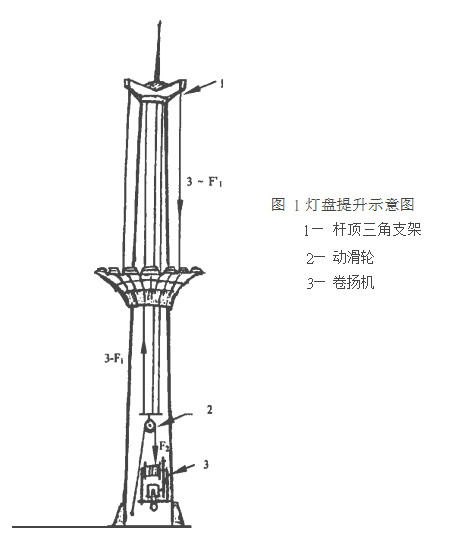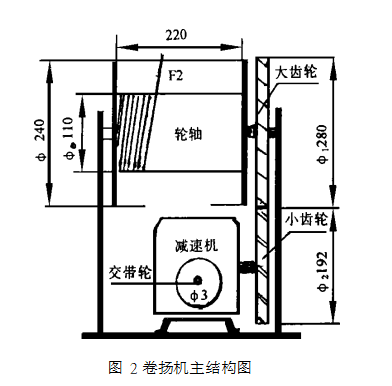0514-84242547# 30m高杆灯灯盘提升系统动力传递的设计计算原理

1、灯盘的提升方法

2、动力传递的设计计算

2. 1 三根分钢索上作用力分别为 F1:

F1 = ( 460÷ 3) /( 0. 93× 0. 93) × 9. 8= 1 737N

2. 2 跨过提升铁板的主钢索盘绕在卷扬机组轮轴的一段受到的作用力 F2:

F2 =3F1 /Z = 23× 1 737 /0. 93= 2 802 N 〈 注 3 〉22. 3 主钢索选用直径为 7. 7m m, 横截面积为22. 37m m2 , 抗 拉 强 度 155kg /m m2 , ( 即 1 519N / m m2 ) ,能承受最大拉力 33 908N。 现提升拉力 F2= 2 802N. 使用中的安全系数 β=  33 908 /2 802=  12. 1

>8。 完全符合国家有关部门规定。

2. 4 卷扬机的主体结构和关键尺寸见图 2。 卷扬机组的轮轴直径 0 = 110m m,可排绕主钢索的长度 L= 215m m。 30m高杆灯,灯盘上升或下降的最大行程为 28m。 主钢索在轮轴上盘绕部分的总长度,即为主钢索跨过动滑轮移动的长度 S= 56m。 据计算: 56m 主钢索在轮轴上需排绕 6层。 排绕第 1层时,轮轴加钢索的力学计算直径 O′0  =  110+   7. 7=  117. 7m m; 排绕第 6层时, 轮轴加钢索后的力学计算直径 O″0 为 190mm。 设这两种情况时,轮轴需付出的最小、最大扭矩分别为 M小 和 M大:

M小=   F2·  ′0   /2=  2 802× 0. 118 /2=  165. 3Nm( ′0=  117. 7mm  0. 118m )

M大=   F2·  ″0   /2=  2 802× 0. 19 /2=  266. 2Nm2. 5 卷扬机的轮轴与大齿轮同轴心组合。 大齿轮的分度圆直径 1 = 280m m,齿数为 70; 与大齿轮衔接的小齿轮 (组装在减速机的输出轴心上)分度圆直径

2 = 192mm ,齿数为 48。 两个传递齿轮的模数皆为

4。 齿轮间的传递效率 η2 =  0. 95。

2. 6 因大齿轮与轮轴同心组合,大齿轮分度圆周上必须付出的力矩等于 M小 或 M大。设大齿轮分度圆周上承担的最大作用力为 F3:

F =  M1 =  266.  2 0. 281 901. 4N;

2. 7 小齿轮与大齿轮衔接,小齿轮必须付出的作用力为 F4 ,付出的力矩 M:

F4=   F3   /η2=  1 901. 4 /0. 95=  2 001. 5N;

M= F4· 2 /2= 2 001. 5× 0. 192 /2= 192. 1Nm

2. 8 若选用 W PA 80型减速机,其输入转速为 800r / min,输出扭矩为 211Nm ,减速比为 62∶ 1,因 211Nm

>M ( 192. 1Nm ) ,符合需求。

2. 9 当减速机的输入转速满足 800r /min(由电动机相应的转速和皮带轮传动比来满足)时,减速机的输出扭矩即为 211Nm ,小齿轮与减速机输出轴同心组合,输送到小齿轮分度圆周上的作用力 F5:

0. 192= 211 /4 > F3 (满足需求)2 = 2 198N> F

2. 10 设暂时选用的电动机为 4P,转速 n1 = 940r / mi n。减速机要求输入转速η2=  800r /mi n〈注 4〉。若电机的输出皮带轮直径 4= 105mm ,则减速机输入皮带轮直径为 3:n1· 43=n2= 940× 105 / 800= 123m m;